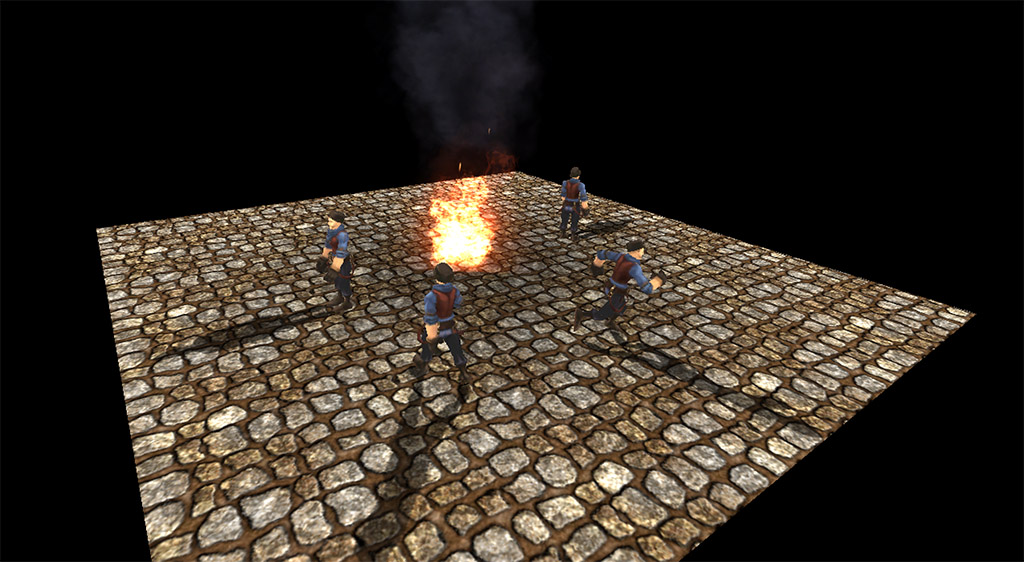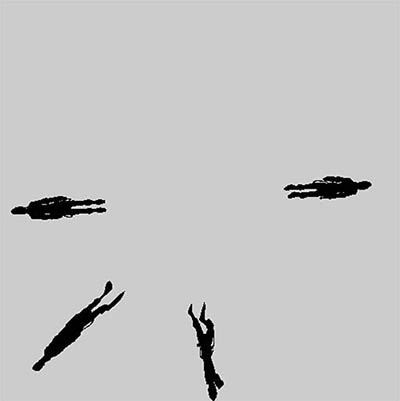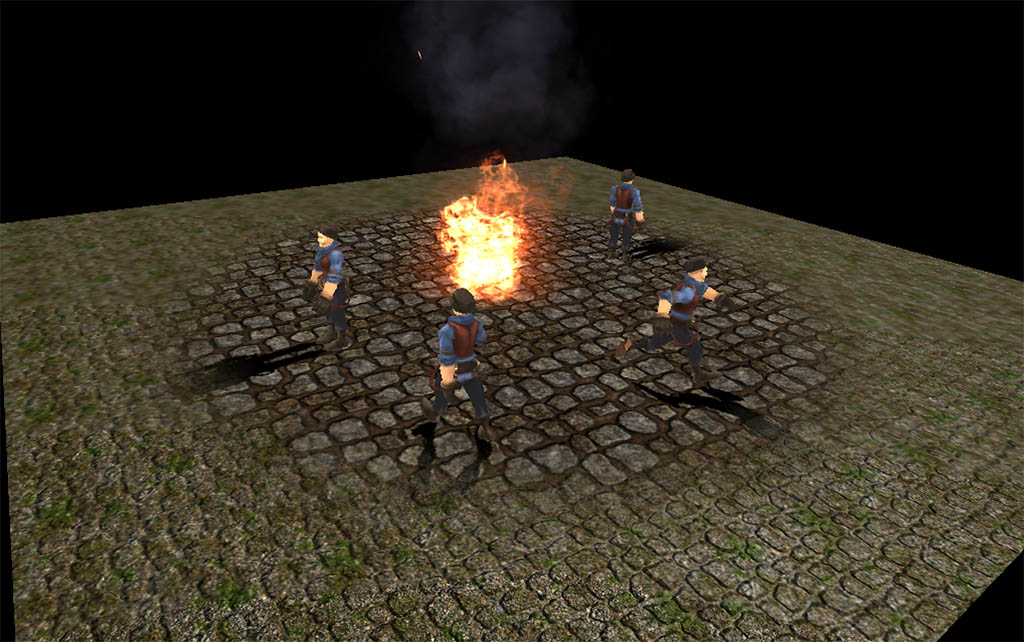# Jim's GameDev Blog# 点阴影

2016-4-7``````// 这段代码每个需要点阴影的角色都需要单独计算
// N 表示的是平面（参考平面方程）。xyz是平面方向的向量，必须是归一化的。w表示沿着前面的xyz方向，从原点偏移多少距离。这里是测试场景，所以写了一个固定值。
Vector4 N = new Vector4(0, 1, 0, 0);
// 点光源光线的方向。
Vector3 L = transform.position - center.position;
L.Normalize();
// 这里就是平面投影矩阵了
Matrix4x4 mat = Matrix4x4.identity;
mat.SetRow(0, new Vector4(N.y * L.y + N.z * L.z, -N.y * L.x, -N.z * L.x, -N.w * L.x));
mat.SetRow(1, new Vector4(-N.x * L.y, N.x * L.x + N.z * L.z, -N.z * L.y, -N.w * L.y));
mat.SetRow(2, new Vector4(-N.x * L.z, -N.y * L.z, N.x * L.x + N.y * L.y, -N.w * L.x));
mat.SetRow(3, new Vector4(0, 0, 0, N.x * L.x + N.y * L.y + N.z * L.z));
// 将矩阵传给模型的材质。注意模型本身是用不到这个矩阵的，是为了下一步渲染到 RenderTexture 做准备。
renderer.material.SetMatrix("_PlanarMat", mat);
``````

``````camera.target = renderTexture;
// 这里还有一步操作A，下面介绍。
// 开始渲染
camera.Render();
``````

``````// 虚拟相机是正交的，所以不需要考虑深度。
Vector3 p0 = new Vector3(0, 0, camera.nearClipPlane);
p0 = camera.ScreenToWorldPoint(p0);
Vector3 p1 = new Vector3(camera.pixelWidth, camera.pixelHeight, camera.nearClipPlane);
p1 = camera.ScreenToWorldPoint(p1);
Vector3 scale = (p0 - p1);
// 这里的plane是Unity内置的，默认1缩放时占世界空间的10个单位。
// 将plane调整为刚好撑满整个虚拟相机拍摄的范围
plane.localScale = new Vector3(Mathf.Abs(scale.x)/10.0f, 1, Mathf.Abs(scale.z) / 10.0f);
``````

``````// vertex
v2f o;
float4 v = i.vertex;
// 需要变换到世界空间，因为平面投影矩阵是在世界空间处理的。
v = mul(_Object2World, v);
v.w = 1;
// 将模型沿着光线方向投射到平面上
v = mul(_PlanarMat, v);
v.xyz /= v.w;
v.w = 1;
// 和以前一样的变换
o.pos = mul(UNITY_MATRIX_VP, v);
return o;
``````